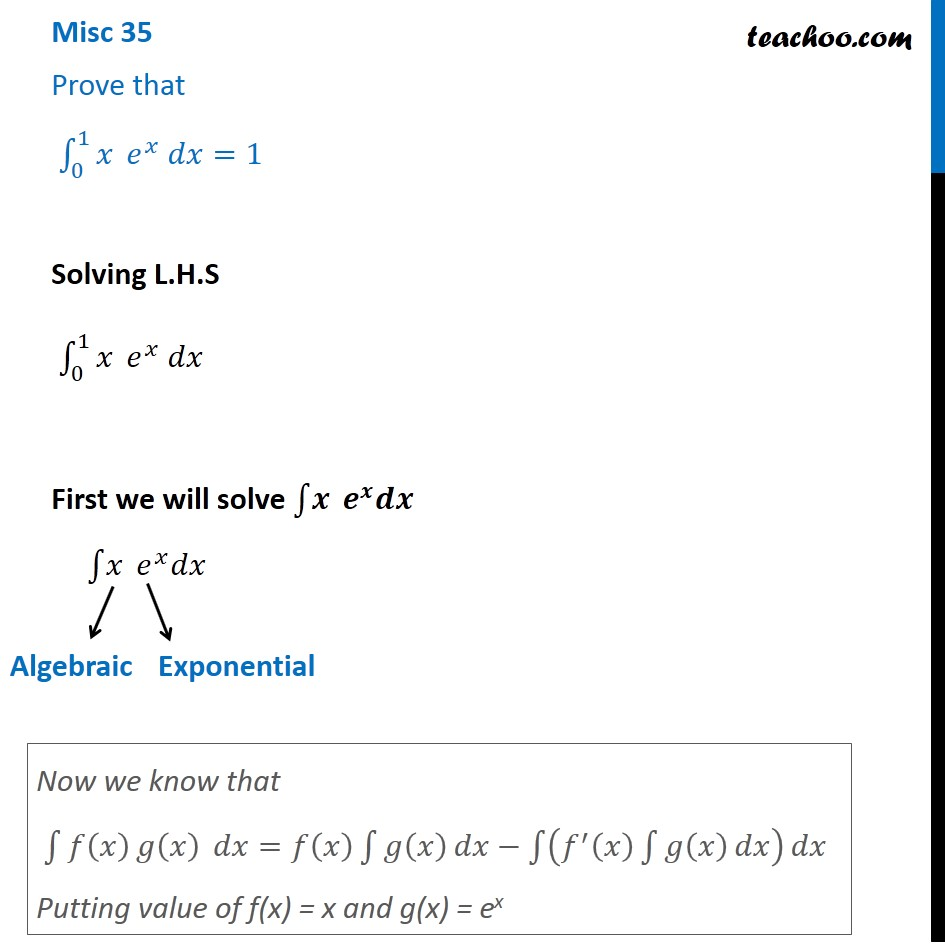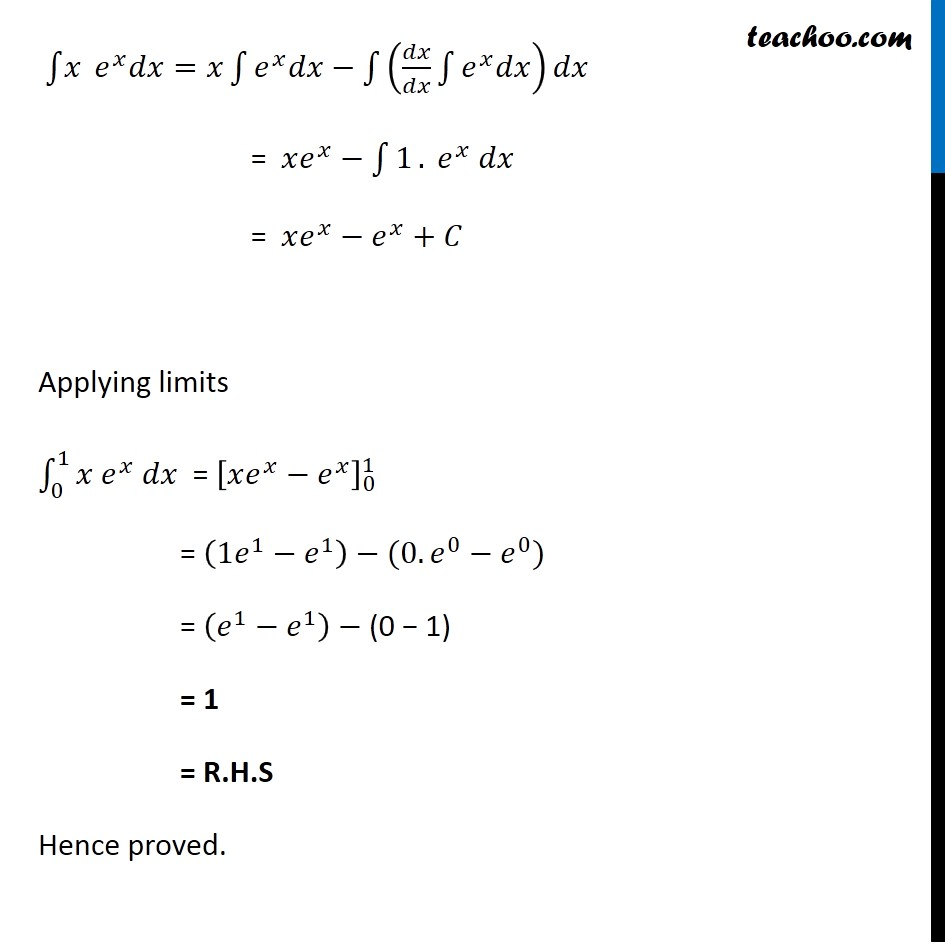Integration Full Chapter Explained - Integration Class 12 - Everything you need1. Chapter 7 Class 12 Integrals
2. Concept wise
3. Definite Integration - By Formulae

Transcript

Misc 35 Prove that ∫_0^1▒𝑥 𝑒^𝑥 𝑑𝑥=1 Solving L.H.S ∫_0^1▒𝑥 𝑒^𝑥 𝑑𝑥 First we will solve ∫1▒𝒙 𝒆^𝒙 𝒅𝒙 ∫1▒𝑥 𝑒^𝑥 𝑑𝑥 Now we know that ∫1▒〖𝑓(𝑥) 𝑔⁡(𝑥) 〗 𝑑𝑥=𝑓(𝑥) ∫1▒𝑔(𝑥) 𝑑𝑥−∫1▒(𝑓^′ (𝑥) ∫1▒𝑔(𝑥) 𝑑𝑥) 𝑑𝑥 Putting value of f(x) = x and g(x) = ex ∫1▒𝑥 𝑒^𝑥 𝑑𝑥=𝑥∫1▒〖𝑒^𝑥 𝑑𝑥〗−∫1▒(𝑑𝑥/𝑑𝑥 ∫1▒〖𝑒^𝑥 𝑑𝑥〗) 𝑑𝑥 = 𝑥𝑒^𝑥−∫1▒1. 𝑒^𝑥 𝑑𝑥 = 𝑥𝑒^𝑥−𝑒^𝑥+𝐶 Applying limits ∫1_0^1▒〖𝑥 𝑒^𝑥 𝑑𝑥〗 = [𝑥𝑒^𝑥−𝑒^𝑥 ]_0^1 = (1𝑒^1−𝑒^1 )−(0.𝑒^0−𝑒^0) = (𝑒^1−𝑒^1 )− (0 − 1) = 1 = R.H.S Hence proved.

Definite Integration - By Formulae

About the AuthorDavneet Singh
Davneet Singh is a graduate from Indian Institute of Technology, Kanpur. He has been teaching from the past 9 years. He provides courses for Maths and Science at Teachoo.